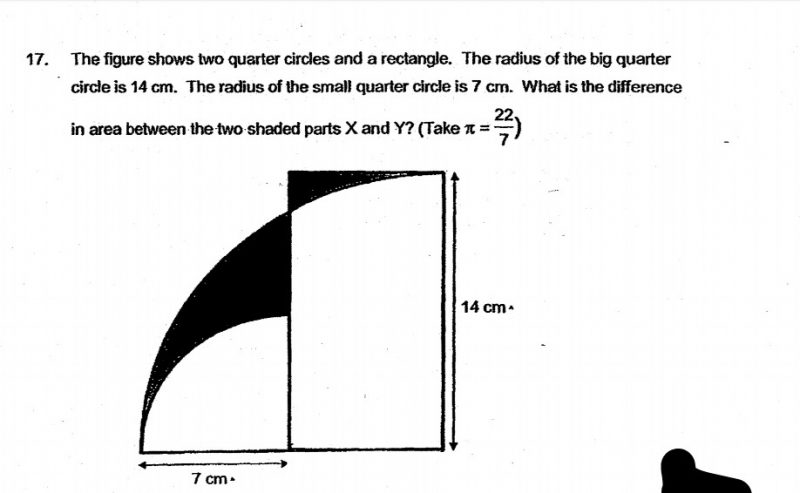# QuestionRegards,

DNLNMUM

Extend the whole figure into a square of (14×14) cm2

Let the new added area be A (top left corner)

Let larger shaded area be X

Let smaller shaded area be Y

Total area of square = (14 x 14) cm2 = 196 cm2

Area of top 2 portions (A + Y) = 196 cm2 – (22/7  x 14 x 14)/4 cm2 = 42 cm2

A = (42 – Y ) cm2

Area of left rectangle = (14 x 7) cm2 = 98 cm2

Area of top 2 portions of left rectangle (A + X) = 98 cm2 – (22/7 x 7 x7)/4 cm2 = 59.5 cm2

A = (59.5-X) cm2

42 – Y = 59.5 -X

X-Y = (59.5 – 42) cm2 = 17.5 cm2